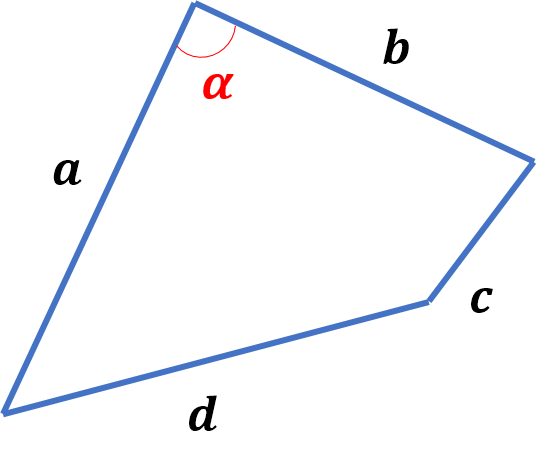# Problem 50464. Calculate convex quadrilateral Area: sides and an angle given

Calculate convex quadrilateral Area: sides and an angle [degree] are given as follows:Round the answer to four decimal places

### Solution Stats

42.31% Correct | 57.69% Incorrect
Last Solution submitted on Apr 30, 2023

### Community Treasure Hunt

Find the treasures in MATLAB Central and discover how the community can help you!

Start Hunting!